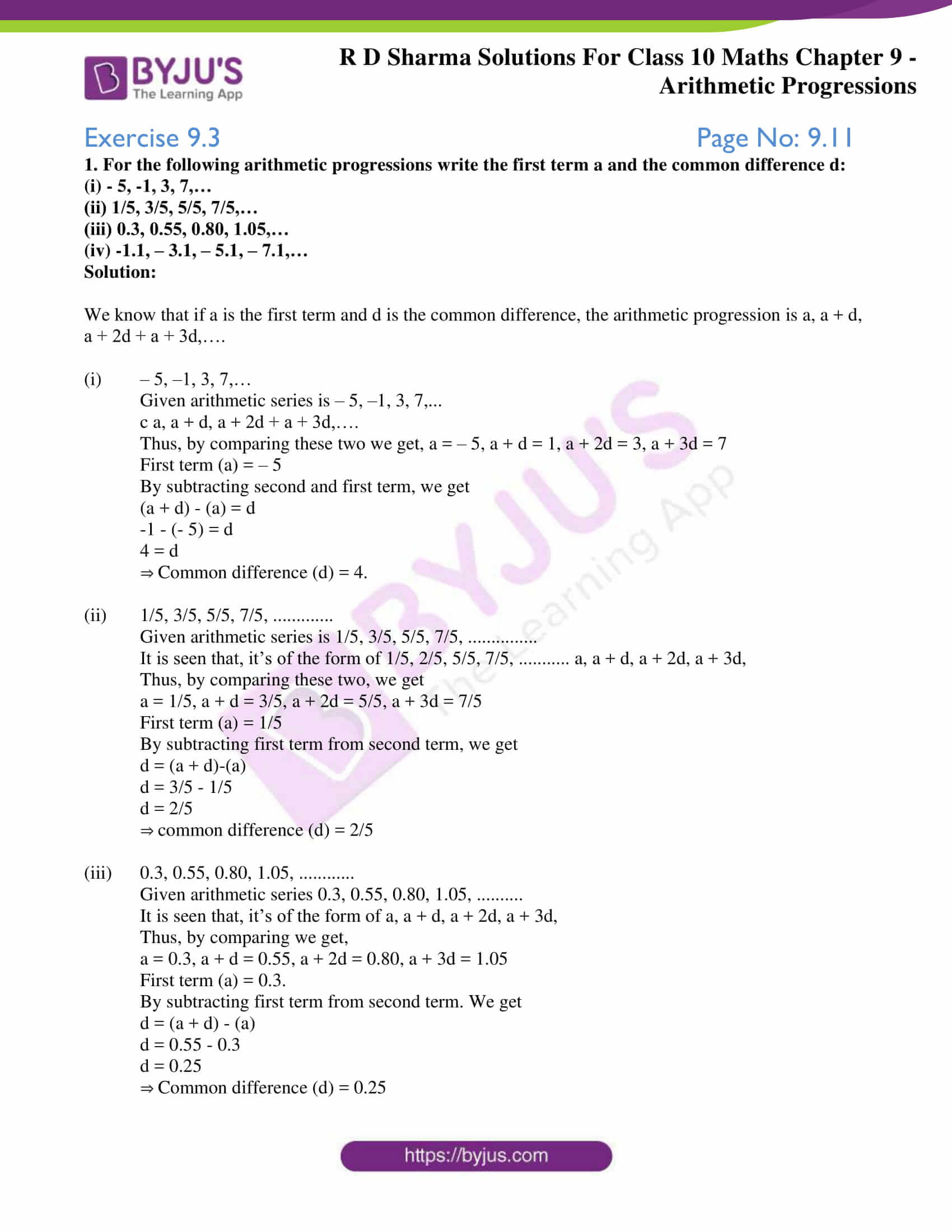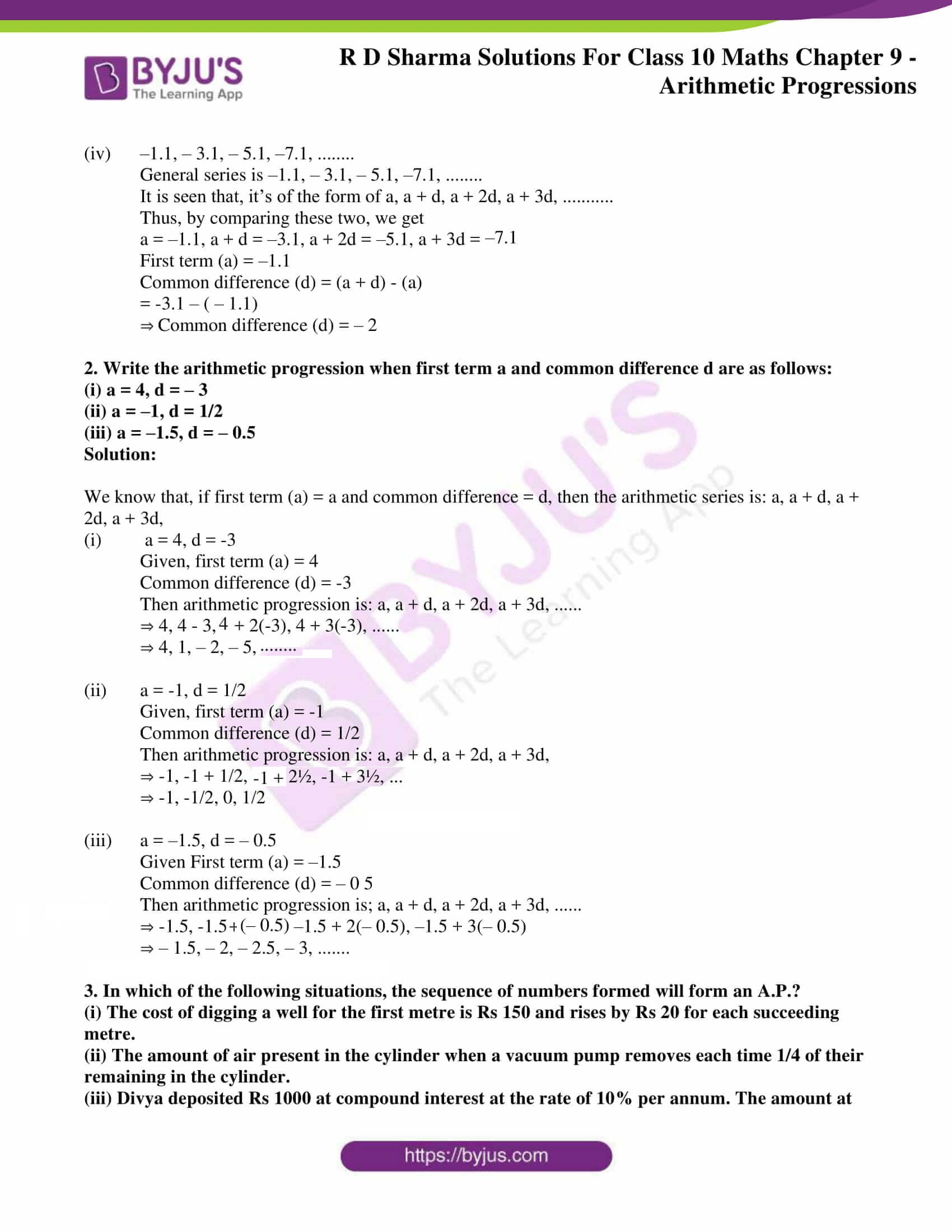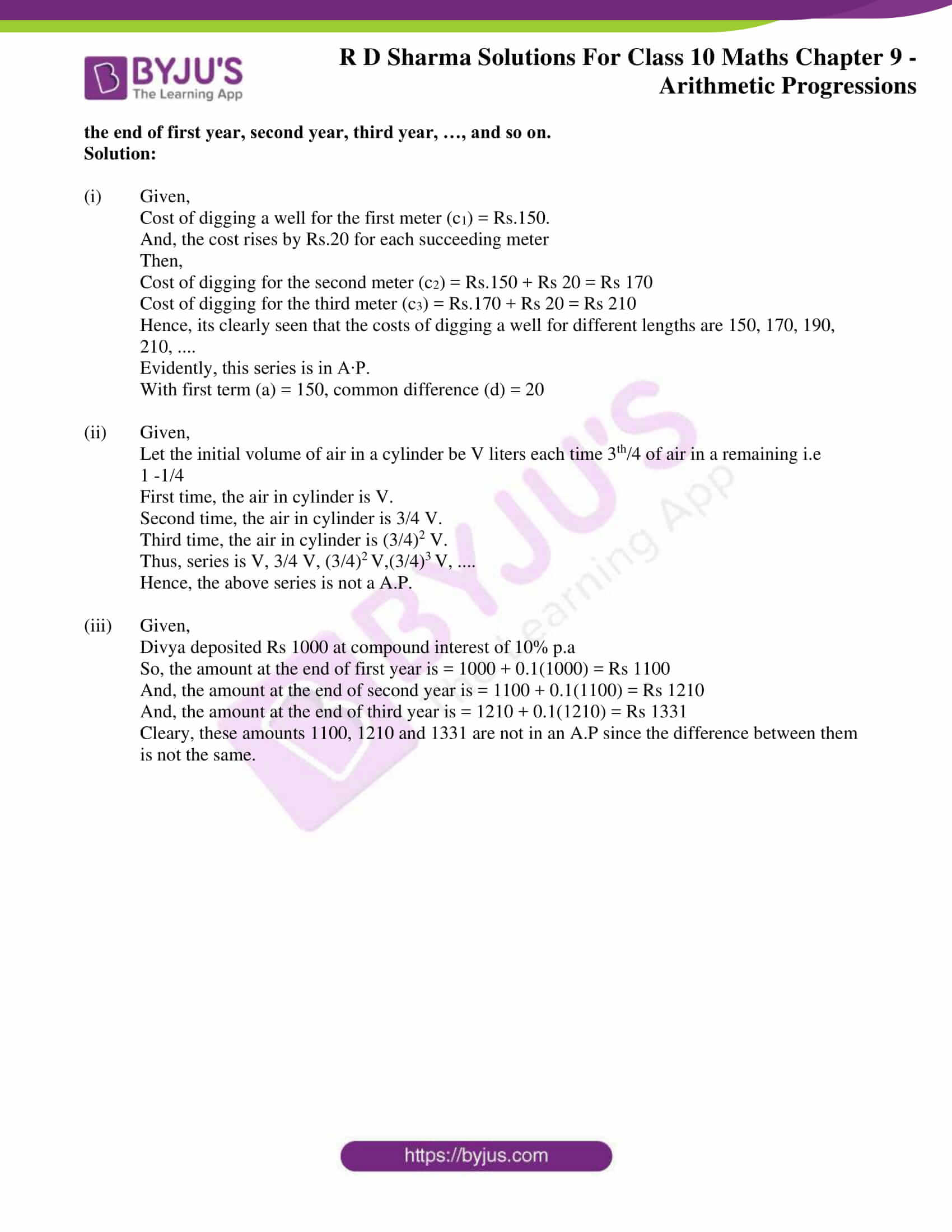# RD Sharma Solutions for Class 10 Maths Chapter 9 Arithmetic Progressions Exercise 9.3

The basic concept of A.P is further tested in this exercise. Problems of finding the common difference and the nth term of the A.P is throughly practised here. The RD Sharma Solutions Class 10 prepared by subject experts at BYJU’S is a very useful resource for students for reference and preparation of exams. Also, download RD Sharma Solutions for Class 10 Maths Chapter 9 Arithmetic Progressions Exercise 9.3 PDF provided below for detailed solutions to problems.

## RD Sharma Solutions for Class 10 Maths Chapter 9 Arithmetic Progressions Exercise 9.3 Download PDF### Access RD Sharma Solutions for Class 10 Maths Chapter 9 Arithmetic Progressions Exercise 9.3

1. For the following arithmetic progressions write the first term a and the common difference d:

(i) – 5, -1, 3, 7,…

(ii) 1/5, 3/5, 5/5, 7/5,…

(iii) 0.3, 0.55, 0.80, 1.05,…

(iv) -1.1, – 3.1, – 5.1, – 7.1,…

Solution:

We know that if a is the first term and d is the common difference, the arithmetic progression is a, a + d, a + 2d + a + 3d,….

(i) – 5, –1, 3, 7,…

Given arithmetic series is – 5, –1, 3, 7,…

c a, a + d, a + 2d + a + 3d,….

Thus, by comparing these two we get, a = – 5, a + d = 1, a + 2d = 3, a + 3d = 7

First term (a) = – 5

By subtracting second and first term, we get

(a + d) – (a) = d

-1 – (- 5) = d

4 = d

⇒ Common difference (d) = 4.

(ii) 1/5, 3/5, 5/5, 7/5, ………….

Given arithmetic series is 1/5, 3/5, 5/5, 7/5, ……………

It is seen that, it’s of the form of 1/5, 2/5, 5/5, 7/5, ……….. a, a + d, a + 2d, a + 3d,

Thus, by comparing these two, we get

a = 1/5, a + d = 3/5, a + 2d = 5/5, a + 3d = 7/5

First term (a) = 1/5

By subtracting first term from second term, we get

d = (a + d)-(a)

d = 3/5 – 1/5

d = 2/5

⇒ common difference (d) = 2/5

(iii) 0.3, 0.55, 0.80, 1.05, …………

Given arithmetic series 0.3, 0.55, 0.80, 1.05, ……….

It is seen that, it’s of the form of a, a + d, a + 2d, a + 3d,

Thus, by comparing we get,

a = 0.3, a + d = 0.55, a + 2d = 0.80, a + 3d = 1.05

First term (a) = 0.3.

By subtracting first term from second term. We get

d = (a + d) – (a)

d = 0.55 – 0.3

d = 0.25

⇒ Common difference (d) = 0.25

(iv) –1.1, – 3.1, – 5.1, –7.1, ……..

General series is –1.1, – 3.1, – 5.1, –7.1, ……..

It is seen that, it’s of the form of a, a + d, a + 2d, a + 3d, ………..

Thus, by comparing these two, we get

a = –1.1, a + d = –3.1, a + 2d = –5.1, a + 3d = –7.1

First term (a) = –1.1

Common difference (d) = (a + d) – (a)

= -3.1 – ( – 1.1)

⇒ Common difference (d) = – 2

2. Write the arithmetic progression when first term a and common difference d are as follows:

(i) a = 4, d = – 3

(ii) a = –1, d = 1/2

(iii) a = –1.5, d = – 0.5

Solution:

We know that, if first term (a) = a and common difference = d, then the arithmetic series is: a, a + d, a + 2d, a + 3d,

(i) a = 4, d = -3

Given, first term (a) = 4

Common difference (d) = -3

Then arithmetic progression is: a, a + d, a + 2d, a + 3d, ……

⇒ 4, 4 – 3, 4 + 2(-3), 4 + 3(-3), ……

⇒ 4, 1, – 2, – 5, – 8 ……..

(ii) a = -1, d = 1/2

Given, first term (a) = -1

Common difference (d) = 1/2

Then arithmetic progression is: a, a + d, a + 2d, a + 3d,

⇒ -1, -1 + 1/2, -1 + 2½, -1 + 3½, …

⇒ -1, -1/2, 0, 1/2

(iii) a = –1.5, d = – 0.5

Given First term (a) = –1.5

Common difference (d) = – 0.5

Then arithmetic progression is; a, a + d, a + 2d, a + 3d, ……

⇒ -1.5, -1.5 + (-0.5), –1.5 + 2(– 0.5), –1.5 + 3(– 0.5)

⇒ – 1.5, – 2, – 2.5, – 3, …….

3. In which of the following situations, the sequence of numbers formed will form an A.P.?
(i) The cost of digging a well for the first metre is Rs 150 and rises by Rs 20 for each succeeding metre.
(ii) The amount of air present in the cylinder when a vacuum pump removes each time 1/4 of their remaining in the cylinder.

(iii) Divya deposited Rs 1000 at compound interest at the rate of 10% per annum. The amount at the end of first year, second year, third year, …, and so on.

Solution:

(i) Given,

Cost of digging a well for the first meter (c1) = Rs.150.

And, the cost rises by Rs.20 for each succeeding meter

Then,

Cost of digging for the second meter (c2) = Rs.150 + Rs 20 = Rs 170

Cost of digging for the third meter (c3) = Rs.170 + Rs 20 = Rs 210

Hence, its clearly seen that the costs of digging a well for different lengths are 150, 170, 190, 210, ….

Evidently, this series is in A∙P.

With first term (a) = 150, common difference (d) = 20

(ii) Given,

Let the initial volume of air in a cylinder be V liters each time 3th/4 of air in a remaining i.e

1 -1/4

First time, the air in cylinder is V.

Second time, the air in cylinder is 3/4 V.

Third time, the air in cylinder is (3/4)2 V.

Thus, series is V, 3/4 V, (3/4)V,(3/4)V, ….

Hence, the above series is not a A.P.

(iii) Given,

Divya deposited Rs 1000 at compound interest of 10% p.a

So, the amount at the end of first year is = 1000 + 0.1(1000) = Rs 1100

And, the amount at the end of second year is = 1100 + 0.1(1100) = Rs 1210

And, the amount at the end of third year is = 1210 + 0.1(1210) = Rs 1331

Cleary, these amounts 1100, 1210 and 1331 are not in an A.P since the difference between them is not the same.